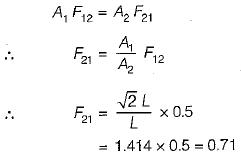# Radiation Heat Transfer - 1

## 10 Questions MCQ Test Topicwise Question Bank for GATE Mechanical Engineering | Radiation Heat Transfer - 1

Description
This mock test of Radiation Heat Transfer - 1 for Mechanical Engineering helps you for every Mechanical Engineering entrance exam. This contains 10 Multiple Choice Questions for Mechanical Engineering Radiation Heat Transfer - 1 (mcq) to study with solutions a complete question bank. The solved questions answers in this Radiation Heat Transfer - 1 quiz give you a good mix of easy questions and tough questions. Mechanical Engineering students definitely take this Radiation Heat Transfer - 1 exercise for a better result in the exam. You can find other Radiation Heat Transfer - 1 extra questions, long questions & short questions for Mechanical Engineering on EduRev as well by searching above.
QUESTION: 1

Solution:
QUESTION: 2

Solution:
QUESTION: 3

### The earth receive at its surface radiation from the sun at the rate of 1400 W/m2. The distance of the centre of sun from the surface of earth is 1.5 x 1011 m and the radius of the sun is 7 x 108 m. Treating the sun as black body, it follows from the above data that sun's surface temperature is about

Solution: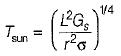where L is mean distance between sun and earth,

r is radius of the sun

Gs is solar constant and equal to 1400 W/m2

σ  is Stefan-Boltzman constan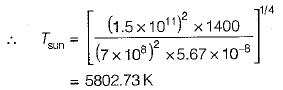QUESTION: 4

What combination of surface characteristics is required for solar collectors?

Solution:
QUESTION: 5

The radiant heat exchange between two bodies depends upon
1. how they see each other
2. their emitting and absorbing characteristics and
3. the medium that intervenes the two bodies

Which of the above statements are valid?

Solution:
QUESTION: 6

Consider the following statements pertaining to emissivity:

1. A gray body has variable monochromatic em issivity, ∈λ, with respect to wavelength .
2. A gray body has a characteristic emissivity value of less than unity which does not vary with temperature.
3. According to kirchoff’s law, the emissivity of the surface of a body is equal to its absorptivity when the body is in thermal equilibrium with its surroundings,
4. Kirchoff’s law holds good for monochromatic radiation.

Which of the above statements are valid?

Solution:
QUESTION: 7

For the same type of shapes , the value of radiation shape factor will be higher when

Solution:
QUESTION: 8

What is the value of shape factor for two infinite* parallel surfaces separated by a distance x?

Solution:
QUESTION: 9

What is the view factor F12 for inclined parallel plates of equal width and a common edge?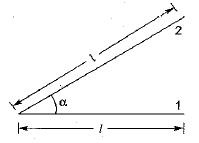Solution:
QUESTION: 10

What is the view factor F21 for the geometry having one side of a diagonal partition within a Long square duet?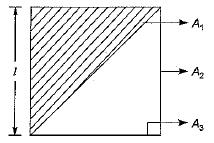Solution: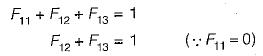By symmetry, F12 = F13 = 0.5